## Thursday, December 29, 2016

### Methods & object class for `phyl.RMA` (phylogenetic RMA regression)

I just added S3 `print`, `residuals`, and `coef` methods for the phylogenetic reduced major axis regression function, `phyl.RMA`. The updates can be seen here.

The following is a quick demo using simulated data:

``````library(phytools)
packageVersion("phytools")
``````
``````##  '0.5.68'
``````
``````tree
``````
``````##
## Phylogenetic tree with 26 tips and 25 internal nodes.
##
## Tip labels:
##  A, B, C, D, E, F, ...
##
## Rooted; includes branch lengths.
``````
``````x
``````
``````##           A           B           C           D           E           F
##  5.02625173  3.84069323  1.40907359  1.62584177 -0.38820852 -0.03660051
##           G           H           I           J           K           L
## -1.15405529 -1.05555766 -1.10500369 -1.82982215 -0.58220778 -0.62621299
##           M           N           O           P           Q           R
##  2.58984857  1.65864863  1.38732527  1.10492094  0.89527230 -1.40154533
##           S           T           U           V           W           X
##  1.23500803 -0.24029572 -0.26507837 -0.77611134 -0.88118355 -2.59262521
##           Y           Z
## -0.11687224 -1.68772212
``````
``````y
``````
``````##           A           B           C           D           E           F
##  5.03364484  4.45525372  2.33034028  1.70260435  1.86376515  0.63005315
##           G           H           I           J           K           L
## -1.27847189 -0.51986100  0.42627759 -0.59603022 -1.02732412 -1.06377260
##           M           N           O           P           Q           R
##  0.11285994  0.08066473 -0.02928941 -0.14296226 -0.38034287 -2.11329071
##           S           T           U           V           W           X
## -0.12823996  1.88409506  2.23634682 -0.82779628 -0.49460318 -2.37103424
##           Y           Z
## -2.07654579 -0.58623675
``````
``````phylomorphospace(tree,cbind(x,y),node.size=c(0,0))
points(cbind(x,y),cex=1.2,pch=21,bg="grey")
``````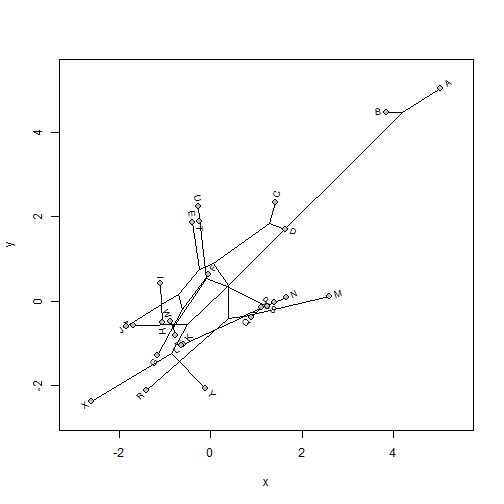Now let's run the RMA regression:

``````obj<-phyl.RMA(x,y,tree)
## print
obj
``````
``````##
## Coefficients:
## (Intercept)           x
##  -0.0424625   1.0647447
##
## VCV matrix:
##          x        y
## x 1.504905 1.119709
## y 1.119709 1.706083
##
## Model for the covariance structure of the error is "BM"
##
## Estimates (or set values):
##    lambda    log(L)
##   1.00000 -69.49404
##
## Hypothesis test based on Clarke (1980; Biometrika):
##        r2         T        df         P
##  0.488316  0.429650 21.290154  0.671767
##
## Note that the null hypothesis test is h0 = 1
``````
``````coef(obj)
``````
``````## (Intercept)           x
##  -0.0424625   1.0647447
``````
``````residuals(obj)
``````
``````##            A            B            C            D            E
## -0.275567308  0.408358649  0.872499213  0.013960519  2.319570602
##            F            G            H            I            J
##  0.711485847 -0.007235186  0.646500875  1.645286860  1.394725642
##            K            L            M            N            O
## -0.364958989 -0.354553159 -2.602204971 -1.642910026 -1.463974066
##            P            Q            R            S            T
## -1.276958414 -1.291116753 -0.578540317 -1.400745657  2.182411146
##            U            V            W            X            Y
##  2.561050105  0.041026621  0.486094798  0.431912090 -1.909644200
##            Z
##  1.253218854
``````

I also wrote a simple `plot` method that throws the RMA line on top of a plotted phylomorphospace. Details can be seen here.

This is what it looks like:

``````plot(obj)
``````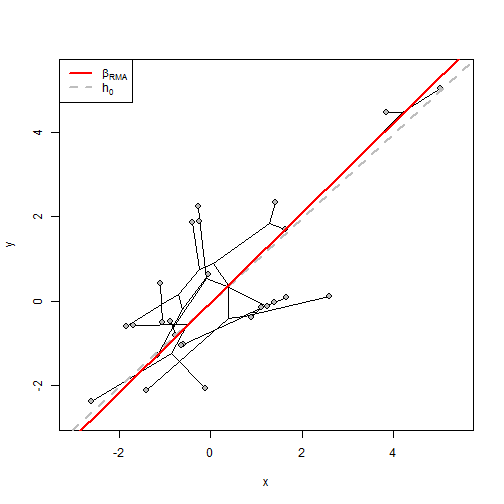We can also flip `x` or `y` just to see what it looks like to plot a negative slope. Note that we can only test a null hypothesis that has the same sign as our fitted RMA line. Here (for fun), I'll test the null hypothesis h0 = -2/3.

``````neg.x<--x
obj<-phyl.RMA(neg.x,y,tree,h0=-2/3)
obj
``````
``````##
## Coefficients:
## (Intercept)           x
##  -0.0424625  -1.0647447
##
## VCV matrix:
##           x         y
## x  1.504905 -1.119709
## y -1.119709  1.706083
##
## Model for the covariance structure of the error is "BM"
##
## Estimates (or set values):
##    lambda    log(L)
##   1.00000 -69.49404
##
## Hypothesis test based on Clarke (1980; Biometrika):
##        r2         T        df         P
##  0.488316  3.206537 21.290154  0.004187
##
## Note that the null hypothesis test is h0 = -0.666666666666667
``````
``````plot(obj)
``````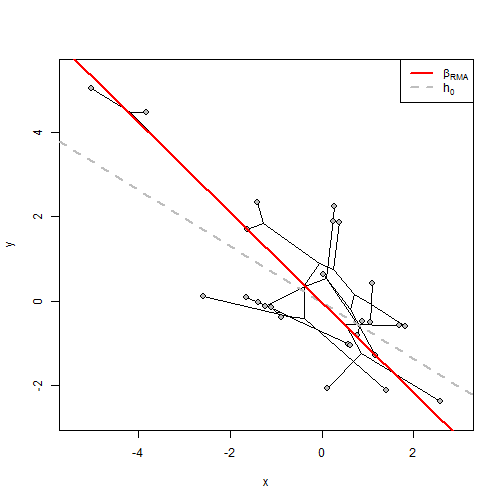That's the idea anyway.

Data for this demo were simulated as follows:

``````library(phytools)
tree<-pbtree(n=26,tip.label=LETTERS)
xy<-fastBM(tree)
x<-xy+fastBM(tree,sig2=0.4)
y<-xy+fastBM(tree,sig2=0.4)
``````

## Tuesday, December 27, 2016

### S3 `as.multiPhylo` method for objects of class `"phylo"`

I just added a tiny update to phytools in which I have included a new S3 method to convert an object of class `"phylo"` to an object of class `"multiPhylo"`.

This is super-simple & looks something like the following:

``````as.multiPhylo.phylo<-function(x,...){
obj<-list(x)
class(obj)<-"multiPhylo"
obj
}

as.multiPhylo<-function(x,...){
if (identical(class(x),"multiPhylo")) return(x)
UseMethod("as.multiPhylo")
}
``````

(along with appropriate `S3method` declaration in `NAMESPACE`, etc.).

The purpose of this is to allow a function to return an object of a consistent class, even if it sometimes returns only one tree. This might be for example, `read.nexus` (in which our input files may contain only 1 phylogeny), or `pbtree`, in which a variable number of phylogenies are to be simulated.

Here is a quick demo of the latter:

``````pbtree(n=26,tip.label=LETTERS)
``````
``````##
## Phylogenetic tree with 26 tips and 25 internal nodes.
##
## Tip labels:
##  A, B, C, D, E, F, ...
##
## Rooted; includes branch lengths.
``````
``````pbtree(n=26,tip.label=LETTERS,nsim=10)
``````
``````## 10 phylogenetic trees
``````

vs.

``````as.multiPhylo(pbtree(n=26,tip.label=LETTERS))
``````
``````## 1 phylogenetic trees
``````
``````as.multiPhylo(pbtree(n=26,tip.label=LETTERS,nsim=10))
``````
``````## 10 phylogenetic trees
``````

The update can be obtained by installing phytools version >= 0.5-67 from GitHub.

## Saturday, December 24, 2016

### Using phytools to plot different genera with different colors

A phytools user asks the following:

“I am following your blog. I have a phylogenetic tree. I would like to color the tree according to their genus name. Could you please help me, providing the code in R?”

There are in fact many ways to plot a tree with edges or clades in different colors. I'm going to give a demo using `paintSubTree` and `plotSimmap` in phytools as follows.

First, let's imagine the following species-level tree in which I have used the syntax Genus_species for all taxon labels.

``````library(phytools)
species.tree
``````
``````##
## Phylogenetic tree with 56 tips and 55 internal nodes.
##
## Tip labels:
##  Rfom_nhijvq, Nvztxu_evipjo, Bswlbtch_azndgw, Ghsygvn_azkqlr, Qptbc_lwcqya, Qptbc_tpnozk, ...
##
## Rooted; includes branch lengths.
``````
``````plotTree(species.tree,ftype="i",fsize=0.7,color="black")
``````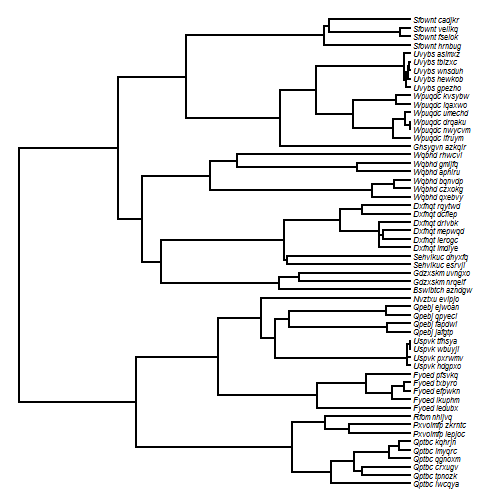First, let's identify all the genera:

``````genera<-sapply(species.tree\$tip.label,function(x) strsplit(x,"_")[])
genera<-sort(unique(genera))
genera
``````
``````##   "Bswlbtch" "Dxfnqt"   "Fyoed"    "Gdzxskm"  "Ghsygvn"  "Nvztxu"
##   "Pxvolmfp" "Qpebj"    "Qptbc"    "Rfom"     "Sehvikuc" "Sfownt"
##  "Uspvk"    "Uvybs"    "Wpuqdc"   "Wqbhd"
``````

Next, find the MRCA of each genus. In the event that the genus contains only one member, we can just paint the terminal edge:

``````for(i in 1:length(genera)){
ii<-grep(genera[i],species.tree\$tip.label)
ca<-if(length(ii)>1)
findMRCA(species.tree,species.tree\$tip.label[ii]) else ii
species.tree<-paintSubTree(species.tree,ca,state=as.character(i),
anc.state="0",stem=TRUE)
}
``````

The following is a trick to remove map segments of zero length:

``````tol<-max(nodeHeights(species.tree))*1e-12
species.tree\$maps<-lapply(species.tree\$maps, function(x,tol)
if(length(x)>1) x[-which(x<tol)] else x,tol=tol)
``````

Now we can set our colors & plot them:

``````cols<-setNames(c("grey",rainbow(length(genera))),
0:length(genera))
plot(species.tree,fsize=0.7,colors=cols,ftype="i",split.vertical=TRUE,
lwd=3,xlim=c(-24,120))
par(font=3)
prompt=FALSE,x=-24,y=24)
``````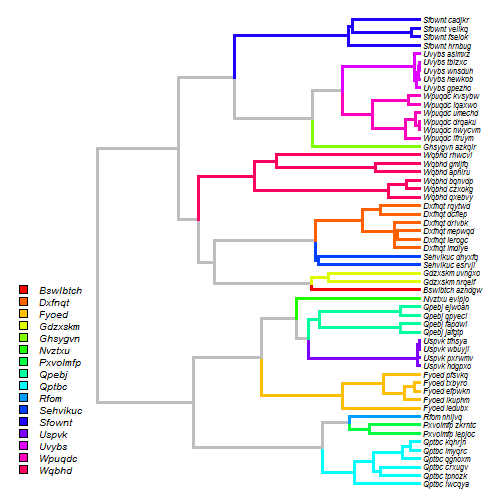That's basically the idea.

The tree for this example is obviously simulated. The following is the code that was used for simulation (taken from a previous post, here)::

``````library(phytools)
## first let's simulate our genus tree:
foo<-function() paste(sample(LETTERS,1),paste(sample(letters,
round(runif(1,min=3,max=7))),collapse=""),sep="")
genera<-replicate(16,foo())
genus.tree<-pbtree(n=length(genera),tip.label=genera,scale=80)
genus.tree\$edge.length[which(genus.tree\$edge[,2]<=Ntip(genus.tree))]<-
genus.tree\$edge.length[which(genus.tree\$edge[,2]<=Ntip(genus.tree))]+20
tips<-c()
for(i in 1:Ntip(genus.tree)){
n.genus<-sample(1:6,1)
if(n.genus>0) for(j in 1:n.genus)
tips<-c(tips,paste(genus.tree\$tip.label[i],
paste(sample(letters,6),collapse=""),sep="_"))
}